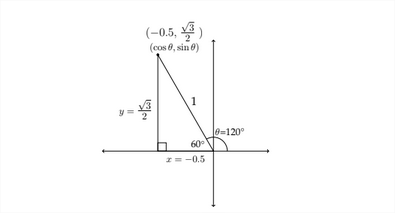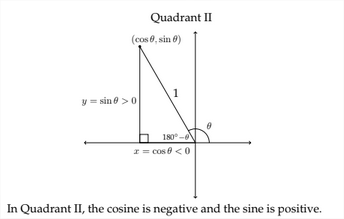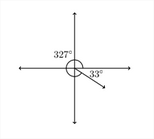# 9.1: Trigonometric Functions of Non-Acute Angles

$$\newcommand{\vecs}{\overset { \rightharpoonup} {\mathbf{#1}} }$$ $$\newcommand{\vecd}{\overset{-\!-\!\rightharpoonup}{\vphantom{a}\smash {#1}}}$$$$\newcommand{\id}{\mathrm{id}}$$ $$\newcommand{\Span}{\mathrm{span}}$$ $$\newcommand{\kernel}{\mathrm{null}\,}$$ $$\newcommand{\range}{\mathrm{range}\,}$$ $$\newcommand{\RealPart}{\mathrm{Re}}$$ $$\newcommand{\ImaginaryPart}{\mathrm{Im}}$$ $$\newcommand{\Argument}{\mathrm{Arg}}$$ $$\newcommand{\norm}{\| #1 \|}$$ $$\newcommand{\inner}{\langle #1, #2 \rangle}$$ $$\newcommand{\Span}{\mathrm{span}}$$ $$\newcommand{\id}{\mathrm{id}}$$ $$\newcommand{\Span}{\mathrm{span}}$$ $$\newcommand{\kernel}{\mathrm{null}\,}$$ $$\newcommand{\range}{\mathrm{range}\,}$$ $$\newcommand{\RealPart}{\mathrm{Re}}$$ $$\newcommand{\ImaginaryPart}{\mathrm{Im}}$$ $$\newcommand{\Argument}{\mathrm{Arg}}$$ $$\newcommand{\norm}{\| #1 \|}$$ $$\newcommand{\inner}{\langle #1, #2 \rangle}$$ $$\newcommand{\Span}{\mathrm{span}}$$$$\newcommand{\AA}{\unicode[.8,0]{x212B}}$$

2.1 Trigonometric Functions of Non-Acute Angles
In Chapter $$1,$$ we learned about the trigonometric functions of positive acute angles that occur within right triangles. If we wish to extend the definition of the trigonometric functions, then we need to define how to determine the values for the sine and cosine of other angles. To do this, consider a right triangle drawn on the coordinate axes. The positive acute angle $$\theta$$ will be the angle created between the $$x$$ -axis and the hypotenuse of the triangle. The lengths of the two legs of the triangle will be the $$x$$ and $$y$$ coordinates of a point in the first quadrant.In the picture above we see a triangle in the first quadrant with a hypotenuse of
1. In this situation, the value of $$\sin \theta=\frac{\rho p p}{h y p}=\frac{y}{1}=y,$$ which is just the $$y$$ -coordinate of the point at the top of the triangle. Correspondingly, the value of $$\cos \theta=\frac{a d j}{h y p}=$$ $$\frac{x}{1}=x,$$ or the value of the $$x$$ -coordinate of the same point.

This allows us to find the sine or cosine for what are known as the quadrantal angles - the angles that are multiples of $$90^{\circ} .$$ If we look at the unit circle (the circle with a radius of 1 ), then we can see the values of the sine and cosine for these angles.In the previous diagram, we see the values for the sine and cosine of the quadrantal angles:
$\cos 0^{\circ}=1 \quad \cos 90^{\circ}=0 \quad \cos 180^{\circ}=-1 \quad \cos 270^{\circ}=0$ $$\sin 0^{\circ}=0 \quad \sin 90^{\circ}=1 \quad \sin 180^{\circ}=0 \quad \sin 270^{\circ}=-1$$
If we take a radius of length 1 and rotate it counter-clockwise in the coordinate plane, the $$x$$ and $$y$$ coordinates of the point at the tip will correspond to the values of the cosine and sine of the angle that is created in the rotation. Let's look at an example in the second quadrant. If we rotate a line segment of length 1 by 120 ", it will terminate in Quadrant II.In the diagram above we notice several things. The radius of length 1 has been rotated by $$120^{\text {ento }}$$ Quadrant II. If we then drop a perpendicular line from the endpoint of the radius to the $$x$$ -axis, we create a triangle in Quadrant II. Notice that the angle supplementary to $$120^{\circ}$$ appears in the triangle and this allows us to find the lengths of the sides of the triangle and hence the values for the $$x$$ and $$y$$ coordinates of the point at the tip of the radius.

Whenever an angle greater than $$90^{\circ}$$ is created on the coordinate axes, simply drop a perpendicular to the $$x$$ -axis. The angle created is the reference angle. The values of the trigonometric functions of the angle of rotation and the reference angle will differ only in their sign $$(+,-) .$$ On the next page are examples for Quadrants $$\mathbb{1}$$,
III, and IV.The process for finding reference angles depends on which quadrant the angle terminates in.
Examples
Find the reference angle for the following angles:
$$1.128^{\circ}$$
$$2.41^{\circ}$$
$$3.327^{\circ}$$
1. An angle of $$128^{\circ}$$ terminates in Quadrant II. To find the reference angle, we would subtract the angle from $$180^{\circ}: \quad 180^{\circ}-128^{\circ}=52^{\circ}$$2. An angle of $$241^{\circ}$$ terminates in Quadrant III. To find the reference angle, we would subtract $$180^{\circ}$$ from the angle: $$\quad 241^{\circ}-180^{\circ}=61^{\circ}$$3. An angle of $$327^{\circ}$$ terminates in Quadrant IV. To find the reference angle, we subtract the angle from $$360^{\circ}: \quad 360^{\circ}-327^{\circ}=33^{\circ}$$Once we know the reference angle, we can find the trigonometric functions for the original angle itself. In example $$1,$$ we had $$128^{\circ},$$ an angle in Quadrant II with a reference angle of $$52^{\circ} .$$ Therefore, if we want to find the sine, cosine and tangent of $$128^{\circ},$$ then we should find the sine, cosine and tangent of $$52^{\circ}$$ and apply the appropriate positive or negative sign.

In Quadrant II, $$x$$ -coordinates are negative and $$y$$ -coordinates are positive. This means that $$\cos \theta<0$$ and $$\sin \theta>0 .$$ The values for this process are given below:
$$\sin 52^{\circ} \approx 0.7880$$
$$\sin 128^{\circ} \approx 0.7880$$
$$\cos 52^{\circ} \approx 0.6157 \quad \cos 128^{\circ} \approx-0.6157$$
$$\tan 52^{\circ} \approx 1.280 \quad \tan 128^{\circ} \approx-1.280^{\circ}$$
In Quadrant III, $$x$$ -coordinates are negative and $$y$$ -coordinates are also negative. This means that $$\cos \theta<0$$ and $$\sin \theta<0 .$$ The values for this process are given below:
$$\sin 61^{\circ} \approx 0.8746 \quad \sin 241^{\circ} \approx-0.8746$$
$$\cos 61^{\circ} \approx 0.4848$$
$$\cos 241^{\circ} \approx-0.4848$$
$$\tan 61^{\circ} \approx 1.8040 \quad \tan 241^{\circ} \approx 1.8040$$
In Quadrant IV, $$x$$ -coordinates are positive and $$y$$ -coordinates are negative. This means that $$\cos \theta>0$$ and $$\sin \theta<0 .$$ The values for this process are given below:
$$\sin 33^{\circ} \approx 0.5446$$
$$\sin 327^{\circ} \approx-0.5446$$
$$\cos 33^{\circ} \approx 0.8387$$
$$\cos 327^{\circ} \approx 0.8387$$
$$\tan 33^{\circ} \approx 0.6494 \quad \tan 327^{\circ} \approx-0.6494$$In Quadrant I, ALL the trigonometric functions are positive. In Quadrant II, the SIN function is positive (as well as the CSC).
In Quadrant III, the TAN function is positive (as well as the COT).
In Quadrant IV, the COS function is positive (as well as the SEC).A common mneumonic device to remember these relationships is the phrase:
"All Students Take Calculus." This can help you remember which trigonometric functions are positive in each of the four quadrants.Reference Angles for Negative Angles
Negatively measured angles rotate in a clockwise direction.There are a variety of methods for finding the reference angle for a negatively valued angle. You can find a positive angle that is co-terminal with the negative angle and then find the reference angle for the positive angle. You can also drop a perpendicular to the $$x$$ -axis to find the reference angle for the negative angle directly.

For example, the angle $$-120^{\circ}$$ terminates in Quadrant III and is co-terminal with the positive angle $$240^{\circ}$$. Either way, when you drop a perpendicular to the $$x$$ -axis, you find that the reference angle is $$60^{\circ} .$$

If you are given the value of one of the trigonometric functions of an angle $$\theta$$ and know which quadrant $$\theta$$ is located in, you can find the other trigonometric functions for that angle.
Example
Given $$\theta$$ in Quadrant $$\mathrm{IV}$$ with $$\cos \theta=\frac{1}{5},$$ find $$\sin \theta$$ and $$\tan \theta$$
If $$\cos \theta=\frac{1}{5},$$ then the adjacent side and the hypotenuse must be in a ratio of $$15 .$$

We can label these sides as 1 and 5 and then find the length of the third side in the triangle. This will allow us to find $$\sin \theta$$ and $$\tan \theta$$Using the Pythagorean Theorem:
13
$$25-10$$
$$x=1$$
we find that the side opposite the reference angle for $$\theta$$ is $$\sqrt{24}$$ or $$2 \sqrt{6}$$. We can now find $$\sin \theta$$ and tan $$\theta:$$
$\sin \theta=\frac{\sqrt{24}}{5}$ and
$\tan \theta=\frac{\sqrt{24}}{1}=\sqrt{24}$

In the problems in this section, the reciprocal functions secant, cosecant and cotangent are used. Remember that:
$\sec \theta=\frac{1}{\cos \theta}=\frac{h y p}{a d y}$ $$\csc \theta=\frac{1}{\sin \theta}=\frac{h y p}{\alpha p p}$$
$$\cot \theta=\frac{1}{\tan \theta}=\frac{a d j}{o p p}$$
Exercises 2.1
Determine the quadrant in which the angle $$\theta$$ lies.
1. $$\cos \theta>0, \tan \theta>0$$
$$\sin \theta<0, \cos \theta>0$$
3. $$\quad \sec \theta>0, \tan \theta<0$$
4. $$\quad \cot \theta>0, \cos \theta<0$$
5. $$\sin \theta>0, \cos \theta<0$$
6. $$\sin \theta>0, \cot \theta>0$$
7. $$\sin \theta<0, \cos \theta<0$$
8. $$\csc \theta>0, \cot \theta<0$$
Determine which quadrant the given angle terminates in and find the reference angle for each.
$$9.195^{\circ}$$
$$10.330^{\circ}$$
$$11.120^{\circ}$$
$$12.210^{\circ}$$
$$13.135^{\circ}$$
$$14.300^{\circ}$$
$$15 . \quad-100^{\circ}$$
$$16.225^{\circ}$$
$$17 . \quad 315^{\circ}$$
$$18 . \frac{5 \pi}{4}$$
19. $$\quad-\frac{2 \pi}{3}$$
$$20 . \quad \frac{7 \pi}{3}$$
$$21 . \quad \frac{11 \pi}{4}$$
$$22 . \quad \frac{7 \pi}{6}$$
23. $$\frac{11 \pi}{6}$$

Find $$\sin \theta, \cos \theta$$ and tan $$\theta$$ in each problem.
24. $$\quad \sin \theta=-\frac{12}{13}, \theta$$ in Quadrant IV
25. $$\quad \cos \theta=-\frac{4}{5}, \theta$$ in Quadrant II
26. $$\quad \cos \theta=\frac{1}{4}, \theta$$ in Quadrant I
27. $$\quad \tan \theta=\frac{3}{2}, \theta$$ in Quadrant III
28. $$\quad \tan \theta=-\frac{4}{5}, \theta$$ in Quadrant II
29. $$\quad \sin \theta=\frac{3}{8}, \theta$$ in Quadrant II
30. $$\quad \sin \theta=-\frac{1}{3}, \theta$$ in Quadrant III
31. $$\quad \tan \theta=5, \theta$$ in Quadrant $$I$$
32. $$\quad \sec \theta=-2, \tan \theta<0$$
33. $$\quad \cot \theta=\sqrt{3}, \cos \theta<0$$
34. $$\quad \tan \theta=-\frac{1}{3}, \sin \theta<0$$
35. $$\quad \csc \theta=\sqrt{2}, \cos \theta>0$$
36. $$\quad \cos \theta=-\frac{2}{5}, \tan \theta>0$$
37. $$\quad \sec \theta=2, \sin \theta<0$$
38. $$\quad \sin \theta=\frac{1}{\sqrt{2}}, \cos \theta>0$$
39. $$\quad \sin \theta=-\frac{2}{3}, \cot \theta>0$$

This page titled 9.1: Trigonometric Functions of Non-Acute Angles is shared under a CC BY-NC-SA 4.0 license and was authored, remixed, and/or curated by Richard W. Beveridge.